Spreadsheets are computer programs that are arranged in a grid format of columns and rows, similar to traditional accounting paper. This tutorial will teach basic concepts of using Excel by creating a spreadsheet calculator that can be very useful when preparing quantity takeoffs and construction estimates.

## Assignment Overview

Create a spreadsheet that matches the appearance of the example and functionality outlined in the assignment scope below.

Your completed spreadsheet calculator should include of the following specifications and functionality.

#### All Sections

• Match the format of the example image as closely as possible. This includes row and column numbers and sizes, color, borders, and text.
• Format the cells to display numbers to 2 decimal places.
• Include custom format displays for all Total cells to indicate the unit of measurement (LF, LY, SF, SY, CF, CY) for the calculation of those cells.
• Create and name one group of user input cells for each section. This is necessary to record a macro and make a functional Clear button.
• Create a button formatted with a macro to clear the content for each individual section.
• Create a single button formatted with a macro to clear the content of the three Area and Volume sections.
• Create a single button formatted with a macro to clear the content of all sections of the spreadsheet.

#### Lineal Feet Calculator Functionality

• Convert inputs from inches and feet to decimal feet in each row.
• Sum the total lineal feet and total lineal yards at the bottom of the section.

#### Rectangle Area and Volume Functionality

• Convert the feet/inch inputs for length, width, and depth to decimal feet, then calculate the perimeter, area, and volume for each row.
• Sum the total of all perimeters, areas, and volumes that are inputted into the cells.

#### Right Triangle Area and Volume Functionality

• Convert the feet/inch inputs for rise, run, and depth to decimal feet, then calculate the hypotenuse, perimeter, area, and volume for each row.
• Sum the total of all perimeters, areas, and volumes that are inputted into the cells.

#### Circular Area and Volume Functionality

• Convert the feet/inch inputs for radius and thickness to decimal feet, then calculate the perimeter, area, and volume for each row.
• Sum the total of all perimeters, areas, and volumes that are inputted into the cells.

#### Totals Section Functionality

• Calculate the total rectangular perimeter, area, and volume in both feet and yards.
• Calculate the total triangular perimeter, area, and volume in both feet and yards.
• Calculate the total circular perimeter, area, and volume in both feet and yards.
• Calculate the sum total or all perimeters, areas, and volumes in both feet and yards.

## Enable the Developer Tool Bar

### How to Enable the Developer Tool Bar

This assignment will require you to set up macros in your spreadsheet. To enable macros, you must enable the Developer Toolbar.

1. Go to the File tab in the top-left corner of Excel.
2. Press the Options button to open the menu.
3. Select Customize Ribbon.
4. Press the checkbox for Developer in the list on the right to enable the toolbar.
5. Press OK.

## Lineal Foot Calculator

The first part of the spreadsheet that will be created is a lineal foot calculator. The length of each segment is entered in feet and inches. This is converted to decimal feet, and the individual sections are totaled and displayed in total lineal feet and total lineal yards. The calculator will also have a clear button which will allow the input to be easily cleared out and different input entered in the calculator (Figure 2-22). This can be very useful in estimating, like helping you calculate how many lineal feet of a particular wall style are in a project.

### Step 01: Set Column Widths

1. Select Column A and adjust it a little narrower so that it doesn’t take as much space on the page.
• Zoom in to the line between column A and B. As the cursor is placed on the dividing line, the cursor changes shape, as is shown in Figure 2-23.
• With the cursor a different shape, click the left mouse button and drag the border line to change the width of the column to approximately two pixels wide.

### Step 02: Creating Borders around Lineal Foot Calculator

1. Create a border around the cells that will be used for the calculator.
• Place the mouse cursor in Cell B6 and drag down and to the right while holding the left mouse button pressed until all the cell between B6 and D38 are highlighted.
• With those cells highlighted, click Home → Font → Borders → All Borders from the Ribbon. The group of cells should display with thin borders between each cell (Figure 2-24).
1. Place a thick border around the B6 to D38 group of cells and the group of cells between B2 and D5.
• Select the group of cells between B6 and D38 and select Home → Font → Borders → Thick Box Border from the Ribbon.
• Select Cells B2 to B5 and repeat the procedure by selecting Home → Font → Borders → Thick Box Border from the Ribbon. The cells should display with a thick border around them (Figure 2-25).

The next step will be to merge several cells in the header of the Lineal Foot Calculator to form larger single cells. A fill color will then be added to the header. Finally, text will be added for titles to the calculator.

1. Select cells B2 to D3, written as B2:D3. With cells B2:D3 highlighted, select Home → Alignment → Merge & Center → Merge & Center from the Ribbon. The six cells will combine into a single large cell (Figure 2-26).
1. Select cells B4:D4. With cells B4:D4 highlighted, select Home → Alignment → Merge & Center → Merge & Center from the ribbon. The three cells should be merged into a single cell (Figure 2-27).
1. Highlight cells B2:D5 and select Home → Font → Theme Color from the ribbon.
• Choose a color of your choice from the color palette for the title header section (Figure 2-28).
1. Click in the group of merged cells B4:D4 and type the text “Lineal Feet”.
• The font and display of the text can be modified using any of the standard Microsoft Office text commands available on the Home → Font tab of the Ribbon.
• Modify the font and color to display a font style of your choosing (Figure 2-29).
1. Add Feet, Inches, and Decimal column titles to the calculator header and modify font style to suit your design (Figure 2-30).

### Step 04: Writing the Function to Convert Feet and Inches to a Decimal in Excel

Feet and inch measurements are used very often in the construction process. One of the features of the calculator is that the dimensions can be input in feet and inches and the calculator will convert those numbers into a decimal format. The basic formula for making that conversion is to add the feet component to the inch component and divide by twelve inches per foot. Mathematically the equation to convert one foot six inches into the decimal equivalent of 1.5 feet is described in the following sequence of formulas.

The formula for doing this in Excel is very similar. The added advantage that Excel has is that the input is not limited to numbers only. Excel will also accept the address of a cell as the input for a formula. For example, if the input in cell B6 was 1, and the input in cell C6 was 6, the formula to total the decimal equivalent could be written in cell D6 as =B6+C6/12 (Figure 2-31).

1. Select cell D6 and enter =B6+C6/12.
2. Press enter. It should now total the input from B6 and C6, converting them into a single decimal number.

### Step 05: Copying the Formula into other Cells

Once a formula has been written it can be copied into other cells and used to automate the calculations.

1. Click in cell D6. A highlighted border will display around cell D6 to signify that it is the active cell.
• A small green box will display in the bottom right-hand corner of the cell. This small green box is the fill handle, and can be used to copy the formula into other cells in the column.
2. Move the mouse cursor over the fill handle and wait until the cursor shape changes from a fat cross shape to a thin cross shape (Figure 2- 32).
1. Click the left mouse button and drag down to copy the formula all the way to cell D38 (Figure 2-33).
1. Double click in any of the cells to check whether the formula has been copied correctly.
• Not only has the formula been copied into the cell, but the cells the formula references have updated to match the correct row numbers for each row (Figure 2-34).
1. Shade cells D6 through D38 light gray.
• This is a standard that is often used to provide a visual reference to cells that have a formula in them. That way you can prevent overwriting the formula with manual inputs (Figure 2-35).

### Step 06: Adding Column Totals

Next, add the formulas for totaling the inputs from the calculator as both lineal feet and lineal yards.

1. Click and drag so that both cell C39 and D39 are highlighted.
2. Merge cells C39 and D39 using Home → Alignment → Merge & Center → Merge & Center from the Ribbon (Figure 2-36).
• These cells will be merged together so that there is a bigger cell to display the totals.
1. Repeat the merging for cells C40 and D40.
2. Select the newly created large cell at C39.
3. Add a thick border around them via Home → Font → Borders → Thick Box Borders from the Ribbon (Figure 2-37).
1. Repeat for the large cell at C40.
2. Label cell B39 as “Lineal Feet”
3. Label cell B40 as “Lineal Yards.
4. Click and drag over cells B39 and B40, and align the text to the right.
1. Add the formula for totalling the column figures as lineal feet in cell C39. Type =SUM(D6:D38) and hit enter.
• The SUM formula adds up the numbers for a specified range of cells.
• In Excel formulas, ranges, conditions, arguments, subordinate functions, etc. are entered between parentheses.
• Note that this is not the only way to enter a formula in C39 that will total up the numbers from D6 to D38. For example, you can use the Insert Function button in the cell editing field, then choose your desired function (Figure 2-41).
• While multiple methods will work, directly entering the function by typing it is the most efficient (Figure 2-44).
1. Add the formula for totalling the column figures as lineal yards in cell C40.
• 3 feet is equal to 1 yard, so to convert a number expressed in feet, you only need to divide it by 3.
• There are several ways to enter a formula that will take the total number of decimal feet in cells D6 to D38 and convert it to a single number of decimal yards.
• One option is to repeat the formula of =SUM(D6:D38) and add /3 after it. This will calculate the total number of lineal feet, and divide it by 3, resulting in the number of lineal yards. =SUM(D6:D38)/3.
• The simpler way to write the formula is to reference cell C39, which already totals the numbers from D6 to D38, and divide it by 3. This formula would be =C39/3 (Figure 2-49).

### Step 07: Modify the Display Characteristics

Using the formula to convert lineal feet to lineal yards may result in an answer that is multiple decimal places long. In this situation, numbers that are displayed to two decimal places will provide a sufficient level of accuracy.

#### Set the Decimal Places

1. Select the range of cells you want to adjust the decimal places for, in this case, D6 through D38.
2. Change the type of data by selecting the drop-down arrow in the Home → Number format drop-down menu.
• This drop-down contains several data formats, including text, numbers, percentages, currency, etc.
• The correct type of data for these cells in the calculator is a number. (Figure 2-50).
• Changing the data type also changes the display to show two decimal places. This is because the default setting in Excel is to display numbers to two decimal places. This can, however, be changed if needed.
1. Change the Feet column to zero decimal places, and inches to two decimal places.
• Highlight the desired cells and click the Increase Decimal, or Decrease Decimal buttons (Figure 2-51).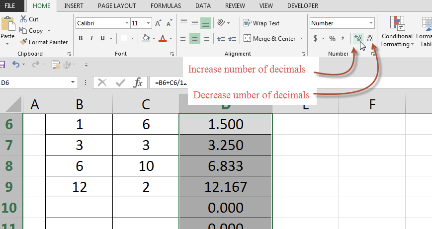Figure 2-51 Buttons can be used to increase or decrease number of decimals to display.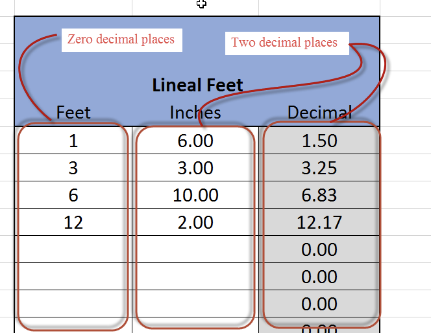Figure 2-52 Change Feet column to zero decimal places and Inches and Decimal columns to two decimal places.

#### Creating a Custom Format Display

1. Display numbers in the total Lineal Feet cell as LF (Lineal Feet) and numbers in the total Lineal Yards cell as LY (Lineal Yards).
• Select the Lineal Feet cell, C39.
• Right-click in the cell and select Format Cells (Figure 2-53).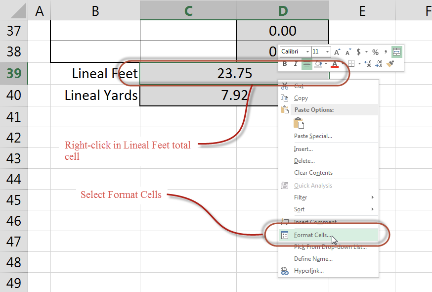Figure 2-53 Right-click in Lineal Feet total cell and select Format Cells.
• Select the Number tab from the Format Cells dialog box, and change the category from Number to Custom (Figure 2- 54).
• Make sure that 0.00 is the active format and is showing in the Type: box.
• Click in the Type: box and place the cursor directly behind the last zero in the 0.00 number sequence (Figure 2-55).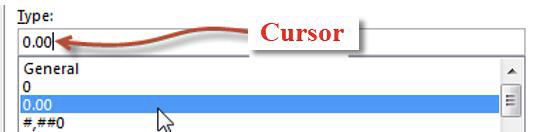Figure 2-55 Place the cursor behind the last zero in the Type box
• Enter a quotation mark, space, and LF, followed by another quotation mark directly behind 0.00 in the Type: box. It should display 0.00” LF” (Figure 2-56).
• Check the formatting in the Sample box to ensure it displays correctly (Figure 2-57).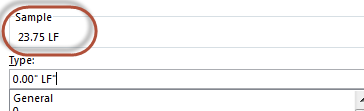Figure 2-57 Sample box shows what the formatting will look like.
• Click OK to save the formatting changes.
• Repeat this procedure for Lineal Yards total cell with the exception that the text should say “LY”.

### Step 08: Create Macros to Clear Data

At this point the calculator is functional. In practice, however, to clear out old inputs for a different set of numbers would require the user to select the numbers in the Feet and Inches columns manually and press the delete key. This can be time-consuming and runs the risk of accidentally selecting cells with formulas in them and deleting the formulas.

To streamline the process of deleting old data, create a macro. A macro is a set of commands that you can program and assign to a button (eg. click a single button to delete the contents of a named group of cells).

#### Creating a Group of Named Cells

1. Select a range of cells; B6 to C38 in this case.
2. Click the Name Box to the left of the function input field, and type LF_Select (Figure 2-59).
1. Press enter. This range of cells is now named LF_Select in the spreadsheet.

There are some rules that must be adhered to when naming cells, or groups of cells. The rules are as follows:

• Each cell, or group of cells must have a unique name which cannot be shared with other cells or Excel macros.
• The name can only be one word long and cannot have any spaces in the name.
• Special characters cannot be used in naming cells or groups of cells.

#### Automating Clearing Calculator Inputs

This group of named cells can now be selected in a mouse click. The content of those cells can then be deleted by single pressing of the Delete key. This is still a two step process, however, and it can be further refined to a single step.

1. Save the file in a macro-enabled format with an xlsm file extension.
• Select File → Save As from the Ribbon.
• Choose a desired location to save your file.
• Give the file a name and select Excel Macro-Enable Workbook (*xlsm) from the list of options in the Save As type box.
• Click the Save button to save the changes.
2. Disable all macros with notifications.
3. Select Developer → Code → Macro Security from the Ribbon. In the Trust Center → Macro Settings dialog box that displays, select the Disable all macros with notifications option (Figure 2-63).
• Macros can be used to break the security of your files, which is why the default is to block them. The step above simply allows you to bypass the safeguard for macros you record in your spreadsheet.
1. Record the LF_Clear macro
• Select Developer → Code → Record Macro from the Ribbon.
• Type in the macro name LF_Clear in the Macro Name cell (Remember, the same name rules apply for naming macros as for naming cells).
• Click Ok to start recording (Figure 2-64).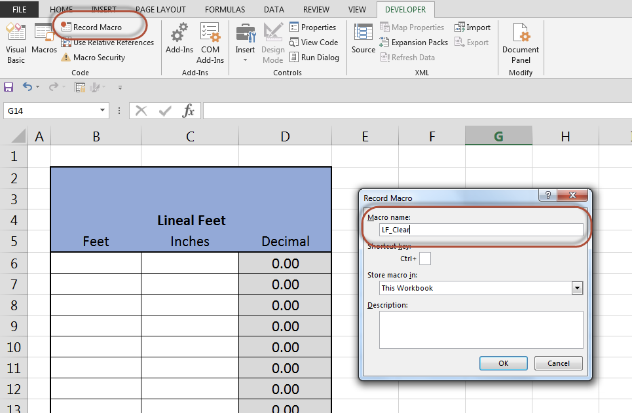Figure 2-64 Select Record Macro and give the macro a name and click OK to start recording the macro.
• Select LF_Select from the drop-down menu in the Name Box (Figure 2-65).
• Press the Delete key on the keyboard.
• Click in the first cell in the named group (B6) so that the calculator is ready to use again.
• Click Developer → Code → Stop Recording in the Ribbon (Figure 2-66).
• Test the macro by selecting Developer → Code → Macro from the Ribbon, then selecting the LF_Clear macro from the list. Click the Run button. The screen should flash briefly and the content of the cells clear (Figure 2-67).
1. Create a Clear Button.
• Select Developer → Controls → Insert → Form Controls → Button (Form Control) from the Ribbon (Figure 2-68).
• After clicking the Button icon, move the cursor over the screen and left-click. This brings up the Assign Macro dialog box. Select the LF_Clear macro and click the Ok button (Figure 2-69).
• Click and drag the button to the desired place on the screen.
• Right-click on the button and select Edit Text (Figure 2-71).
• Change the text of the button to display “Clear”.
• Click somewhere other than the button to exit editing mode.
• Test the Clear button by clicking it. The screen should flash briefly, and the contents of the calculator clear (Figure 2-72).

## Area and Volume Calculators Formatting

The Lineal Feet portion of the spreadsheet calculator should now be fully functional. The area and volume portions of the calculators are completed in a similar fashion.

### How to Format the Area and Volume Calculators

The first step in completing the remainder of the calculator is to format the Excel spreadsheet to display the rectangular area and volume, triangle area and volume, circular area and volume, totals, sections of the calculator. Figure 2-73 shows what the formatting of the finished calculator looks like and can be used as a pattern in preparing your calculator.

## Rectangle Area and Volume Calculator

### Step 01: Enter Formulas in the Rectangle Area and Volume Calculator

Remember that the formulas for perimeter, area, and volume all require specific measurements from the shape for which you calculate those figures. The basic steps for creating these formulas are as follows:

1. Reference the measurement for each length of the shape as a single decimal.
• In Excel syntax, this would be =”feet” + “inches” /12.
• These measurements will be expressed this way in the larger formulas for calculating perimeter, area, and volume.
2. Enter the formula for the specific calculation you want to make.
3. Click and drag the formula to all of the cells in that column.
• This allows you to measure multiple shapes without having reset the calculator.
4. Enter the formula to calculate the sum of all totals in the calculator for both Total Feet and Total Yards.

#### Perimeter Formula Example

1. Perimeter of a rectangle is expressed as 2 x Side 1 + 2 x Side 2.
2. The formula in the spreadsheet calculator would be a little more complicated because the input would need to be converted to decimal form. In addition, there are issues with math order of operations, and so some parentheses will need to be added to the Excel equation.
3. Consider the first row for the Rectangle Area and Volume calculator.
• The perimeter formula will be entered in the merged cell at N5.
• When the calculator is functional, it will take manually entered numbers from the width and length cells and calculate the perimeter.
• The formula for the perimeter of a rectangle in the first row of the Rectangle Area and Volume calculator would be written as follows (Figure 2-75).
• The black parentheses in each half of the equation represent the measurements of length and width converted to decimal feet. Without the parentheses, the calculator would multiply 12 * 2 first because the math order of operations requires multiplication before division. The inches would then be divided by the calculated 24 instead of the proper 12. Figure 2-76 shows the correct formula written in the calculator.
1. The correct formula can then be dragged down to fill the remainder of the calculator.

#### Area Formula Example

1. Area of a rectangle is expressed as Side 1 x Side 2.
2. The formula in the spreadsheet calculator would be a little more complicated because the input would need to be converted to decimal form.
3. Consider the first row for the Rectangle Area and Volume calculator.
• The area formula will be entered in the merged cell at P5.
• When the calculator is functional, it will take manually entered numbers from the width and length cells and calculate the area.
• The formula for the area of a rectangle in the first row of the Rectangle Area and Volume calculator would be written as follows (Figure 2-77).
• As with the perimeter formula, length and width are expressed within parentheses so each measurement will be converted to decimal feet.
1. The correct formula can be dragged down to fill the remainder of the calculator.

#### Volume Formula Example

1. Volume of a rectangular shape is expressed as Length x Width x Depth.
2. The formula in the spreadsheet calculator would be a little more complicated because the input would need to be converted to decimal form.
3. Consider the first row for the Rectangle Area and Volume calculator.
• The volume formula will be entered in the merged cell at R5.
• When the calculator is functional, it will take manually entered numbers from the width, length, and depth cells and calculate the volume.
• The formula for the volume of a rectangular shape in the first row of the Rectangle Area and Volume calculator would be written as =(H5+I5/12)*(J5+K5/12)*(L5+M5/12).
4. The correct formula can be dragged down to fill the remainder of the calculator.

### Step 03: Complete the Rectangle Area and Volume Totals

The final portion of the Rectangle Area and Volume calculator is to complete the totals of the perimeter, area and volume portions of the calculator.

• The Total Feet value of each column is determined by using the SUM function, similar to what was previously learned when completing the Lineal Feet portion of the calculator.
• The Total Yards value for the perimeter is determined by dividing the Total Feet value by three, as was previously done with the Lineal Feet portion of the calculator.
• The totals for the area and volume portions are done in similar fashion.
• Remember that there are nine square feet in a square yard and 27 cubic feet in a cubic yard.
• Figure 2-80 shows the perimeter totals for rectangle area and volume calculations. Use the numbers from this example to check the accuracy of your formulas.
• Custom formats should also be used to identify the correct unit of the total such as FT, YDS, SF, SY, CF, and CY.
• In addition, the custom format should display the total to two decimal places, as was done with the Lineal Feet portion of the calculator.

### Step 04: Complete the Rectangle Clear Macro

The final element to complete with the Rectangle Area and Volume section of the calculator is to create a clear button and a macro to clear this portion of the calculator.

• This is done by following a similar process that was used for the Lineal Feet portion of the calculator. The cells to be cleared will first need to be selected and given a custom name, such as Rect_Select (Figure 2-81).
• Next, a macro is recorded, named Rect_Clear, that selects the Rect_Select named group of cells and deletes the content of the cells.
• Finally, the clear button is assigned to that macro by right clicking on the button and selecting Assign Macro from the context menu (Figure 2-82).

## Right Triangle Area and Volume Calculator

### Step 01: Enter Formulas in the Right Triangle Area and Volume Calculator

#### Right Triangle Area and Volume Formulas

In construction, the sides of a triangle are named run, rise, and hypotenuse, respectively. The programming for the Right Triangle Area and Volume calculator will have manual entries for rise, run, and depth in feet and inches. A formula to calculate the hypotenuse of the triangle is added because that measurement is required to calculate perimeter.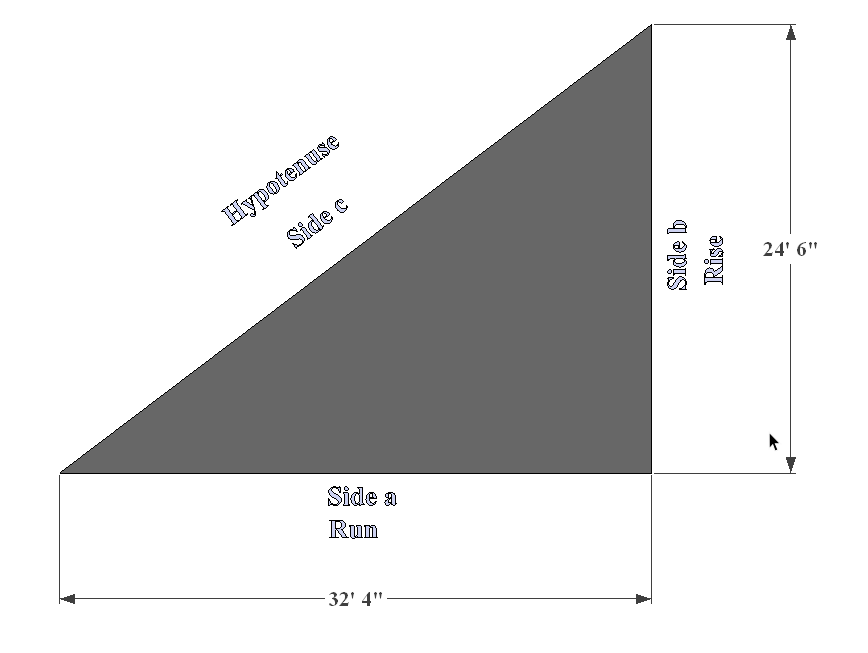Figure 2-83 A right triangle using construction terms for the sides
• The mathematical formula for finding the hypotenuse of a right triangle is known as Pythagorean’s Theorem, which states that the sum of the legs squared is equal to the hypotenuse squared.
• Written mathematically the formula for calculating the hypotenuse of a right triangle is a2 + b2 = c2
• a = Run
• b = Rise
• c = Hypotenuse
• The SQRT (square root) function will be used for your hypotenuse formula in the calculator.
• As in previous examples, the lengths for rise, run, and depth will be expressed using the formula for converting feet and inches to decimal feet.
• Excel uses ^ to express exponents. ^2 = squared, ^3 = cubed, etc.

#### Hypotenuse Formula Example

1. Hypotenuse of a right triangle is expressed as Run squared + Rise squared = Hypotenuse squared.
1. This means you will need to find the square root of whatever c2 equals in order to find the hypotenuse.
1. The formula in the spreadsheet calculator would be a little more complicated because the input would need to be converted to decimal form.
2. Consider the first row for the Right Triangle Area and Volume calculator.
1. The hypotenuse formula will be entered in the cell at M16.
2. When the calculator is functional, it will take manually entered numbers from the run and the rise to calculate the hypotenuse.
3. The formula to find the hypotenuse of a triangle in the first row of the Right Triangle Area and Volume calculator would be written as follows (Figure 2-84).
1. The correct formula can be dragged down to fill the remainder of the calculator.

#### Formulas for Calculating Right Triangle Perimeter, Area, and Volume

Previous instructions in this document outline the procedure for formatting, entering formulas, and creating macros. You have likely noticed that the instructions have become less detailed as they have progressed. The last steps will be completed on your own with minimal guidance from this walkthrough.

• Perimeter = Run + Rise + Hypotenuse
• Area = (Run x Rise) / 2
• Remember order of operations when writing the syntax for this formula in Excel.
• Volume = Area x Depth

Use the manual entries in Figure 2-91 to check the accuracy of your formulas for hypotenuse, perimter, area, and volume.

## Circular Area and Volume Calculator

### Step 01: Enter Formulas in the Circular Area and Volume Calculator

Use previous instructions and the following information to complete the Circular Area and Volume Calculator.

• In a circle, the radius is the length from the center of the circle to any point at the edge.
• A circle contains 360 degrees.
• If you enter anything less than 360 in the degree column, you are indicating that the shape is not a complete circle. You still need this number, though, because some shapes in a construction project will only be partial circles (arches).
• The thickness column refers to the height of a column, not the radius of a sphere. You will use this number when calculating the volume of a circular area.
• Circle Perimeter = 2 x 𝛑 x Radius (2𝛑r)
• Because your calculator must also calculate the perimeter of an arch, you must add a reference to the Angle column. This will be expressed as (“angle degrees” / 360).
• Circle Area = 𝛑r2
• Because your calculator must also calculate the area of an arch, you must add a reference to the Angle column. This will be expressed as (“angle degrees” / 360).
• Pi, or 𝛑, is entered in Excel using the PI function. It is entered as PI().
• When you enter PI() in a formula, you may receive the following dialog box. Click Ok and continue.

#### Example Formulas for Circular Perimeter and Area Using Excel Syntax

Don’t forget to drag down the formulas for the whole columns, enter formulas for total feet and total yards, and create a clear button for this calculator.

## Totals Area of the Calculator

### How to Complete the Totals Section

The Totals portion of the calculator is completed by bringing the individual totals for each calculator section. For example, Figure 2-101 shows how the Total Yards conversion of the circular total cubic yard volume is brought forward by making the cell equal to the individual cell in that portion of the calculator.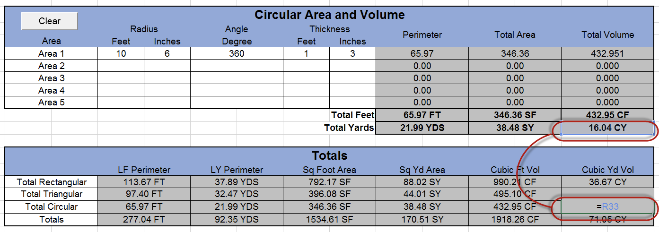Figure 2-101 Transferring information from one cell to another to get totals